Question

# What is the equivalent resistance of each group of resistors shown in the figure below? In...

What is the equivalent resistance of each group of resistors shown in the figure below? In the figure, R1 = 2.0 Ω, R2 = 3.0 Ω, R3 = 8.0 Ω, and R4 = 4.0 Ω. There is no figures present just Group A is in a series with R1 and R2 and R3. The secound Group is in a series with R4 and R4 and R4.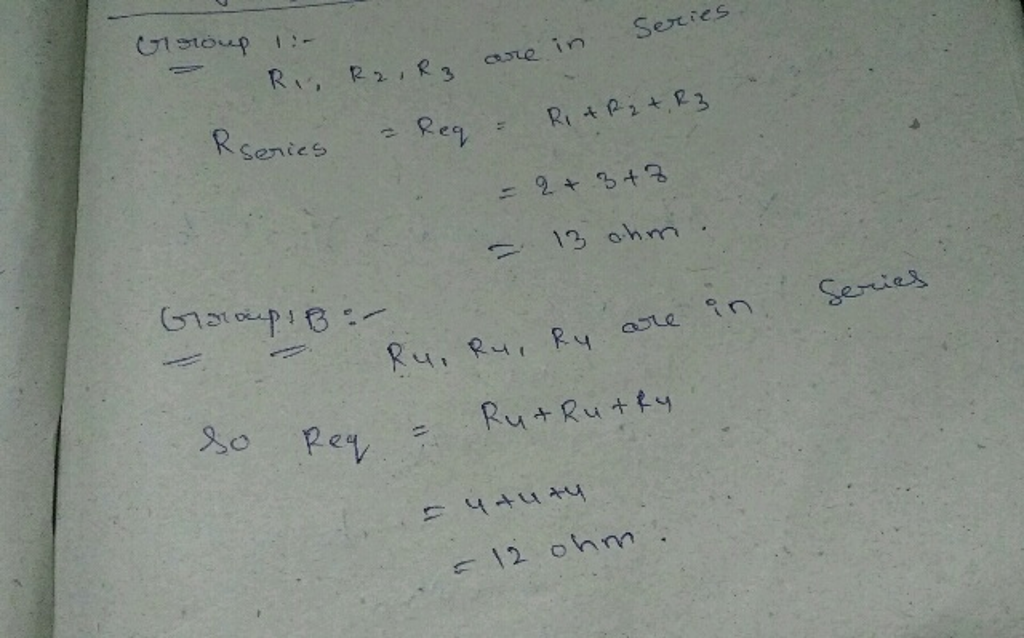#### Earn Coins

Coins can be redeemed for fabulous gifts.

Similar Homework Help Questions
• ### Find the equivalent resistance of the combination of resistors shown above.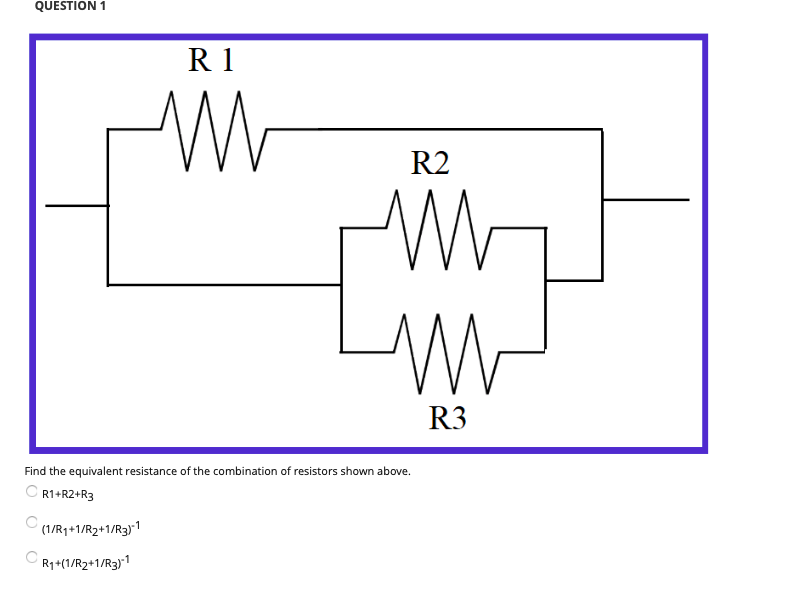Find the equivalent resistance of the combination of resistors shown above.R 1+R 2+R3(1 / R1+1 / R2+1 / R3)^{-1}R1+(1 / R2+1 / R3)^{-1}Find the equivalent resistance of the resistors shown above.(1 / R1+1 / R2)^{-1}+R3+R4R1+R2+(1 / R3+1 / R4)^{-1}R1+R2+R3+R4Assume the equivalent resistance in question 1 was 10 Ω and the equivalent resistance in question 2 was 40 Ω, and the same resistors were used for this new system. Determine the equivalent resistance of this system.

• ### Consider the figure below: a. Find the equivalent resistance of the group of resistors in the...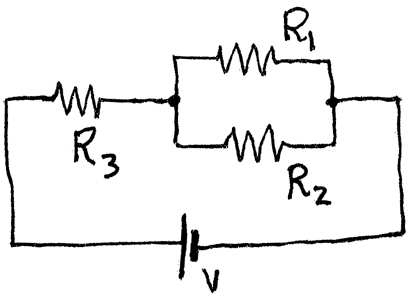Consider the figure below: a. Find the equivalent resistance of the group of resistors in the figure if R1=2630Ω, R2=4890Ω, and R3=2380Ω. b. If these resistors are connected to a V=12.0V battery as shown in the figure, find the potential difference across R2. c. Find the current flowing through R2. 3 2.

• ### A four-resistor circuit is shown in the figure. The values of the resistors are as follows:...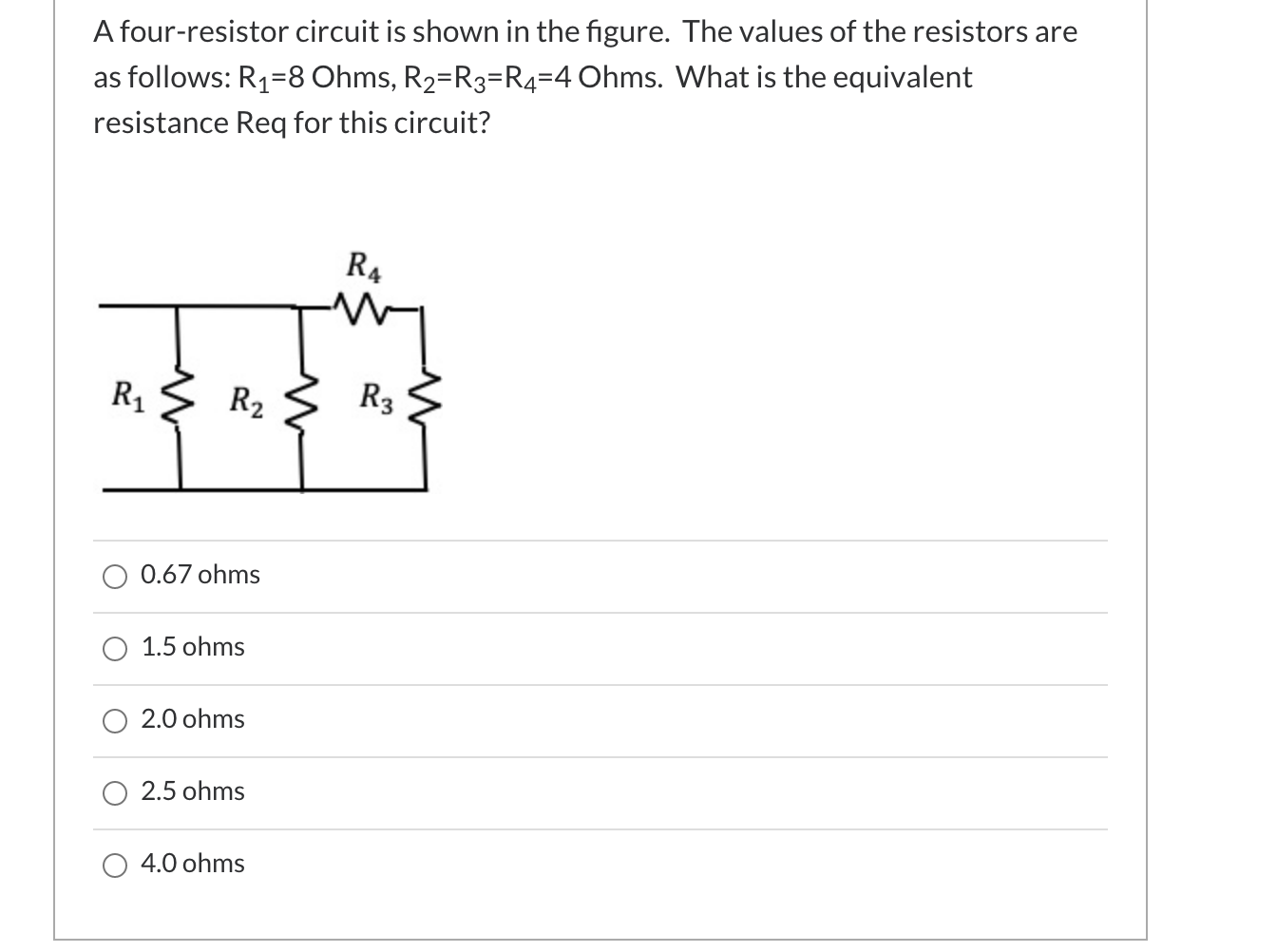A four-resistor circuit is shown in the figure. The values of the resistors are as follows: R1=8 Ohms, R2=R3=R4=4 Ohms. What is the equivalent resistance Req for this circuit? R4 R1 ş R2 R3 0.67 ohms 1.5 ohms 2.0 ohms 2.5 ohms 4.0 ohms

• ### A four-resistor circuit is shown in the figure. The values of the resistors are as follows:...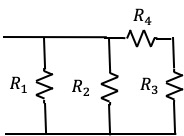A four-resistor circuit is shown in the figure. The values of the resistors are as follows: R1=8 Ohms, R2=R3=R4=4 Ohms. What is the equivalent resistance Req for this circuit? a) 0.67 ohms b) 1.5 ohms c) 2.0 ohms d) 2.5 ohms e) 4.0 ohms R4 w m R1 R2 R3 -

• ### A four-resistor circuit is shown in the figure. The values of the resistors are as follows:...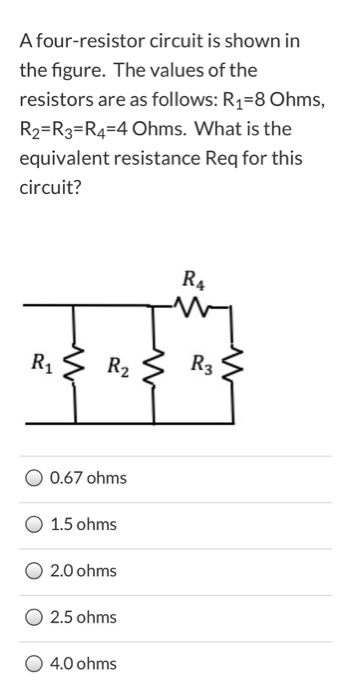A four-resistor circuit is shown in the figure. The values of the resistors are as follows: R1=8 Ohms, R2=R3=R4=4 Ohms. What is the equivalent resistance Req for this circuit? RA R1 R2 R3 0.67 ohms 1.5 ohms 2.0 ohms 2.5 ohms 4.0 ohms

• ### A four-resistor circuit is shown in the figure. The values of the resistors are as follows:...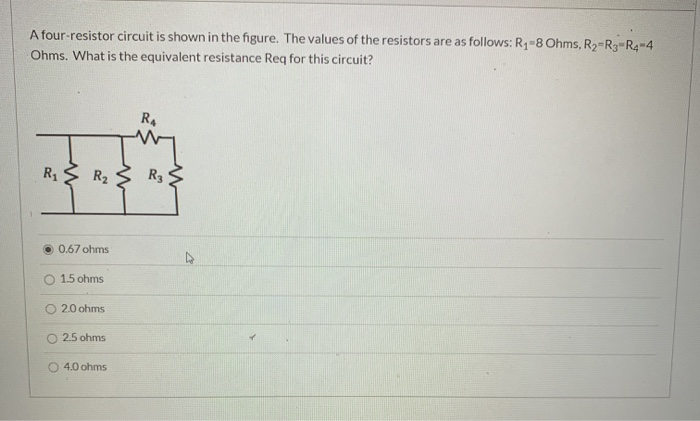A four-resistor circuit is shown in the figure. The values of the resistors are as follows: R1-8 Ohms, R2-R3-R4-4 Ohms. What is the equivalent resistance Req for this circuit? RA R2 R3 0.67 ohms 1.5 ohms O 2.0 ohms O 2.5 ohms 4.0 ohms

• ### 3. Equivalent Resistance (20 pts) 222-152C The resistors in the figure have the following R1 ....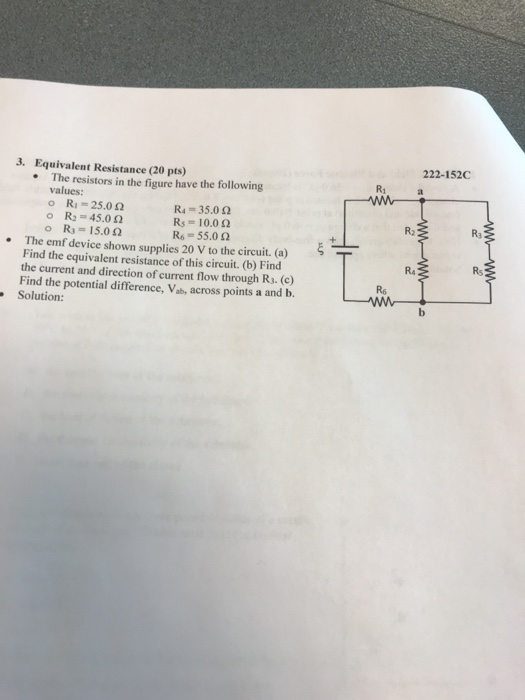3. Equivalent Resistance (20 pts) 222-152C The resistors in the figure have the following R1 . values o Ritz 25.0 Ω o R2-45.0 Ω o R3 15.0 Ω R4-35.0 Ω Rs. 10.0 Ω R6- 55.0 2 R2 Ra The emf device shown supplies 20 V to the circuit. (a) Find the equivalent resistance of this circuit. (b) Find the current and direction of current flow through R3. (c) Find the potential difference, Vab, across points a and b. Solution: ....

• ### A four-resistor circuit is shown in the figure. The values of the resistors are as follows:...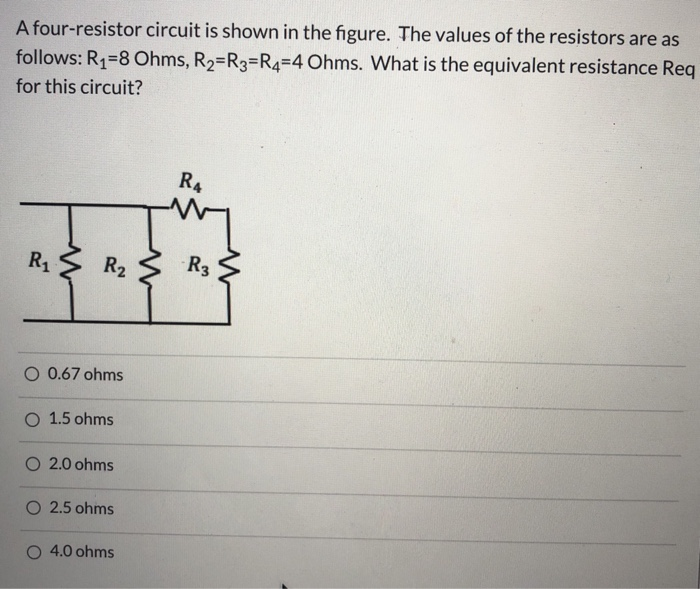A four-resistor circuit is shown in the figure. The values of the resistors are as follows: R1=8 Ohms, R2=R3=R4=4 Ohms. What is the equivalent resistance Req for this circuit? RA R1 R2 -R₃ O 0.67 ohms O 1.5 ohms 2.0 ohms O 2.5 ohms 4.0 ohms

• ### Compute the equivalent resistance of the network in the figure below, and find the current in...

Compute the equivalent resistance of the network in the figure below, and find the current in each resistor. (R1 = 4.00 Ω, R2 = 7.00 Ω, R3 = 10.0 Ω, R4 = 2.00 Ω, and ε = 61.0 V.) The battery has negligible internal resistance. equivalent resistance Incorrect: Your answer is incorrect. Ω current in each resistor Incorrect: Your answer is incorrect. A (Resistor R1) A (Resistor R2) A (Resistor R3) A (Resistor R4)

• ### What is the equivalent resistance of group (a) of resistors shown in the figure? (Figure 1)...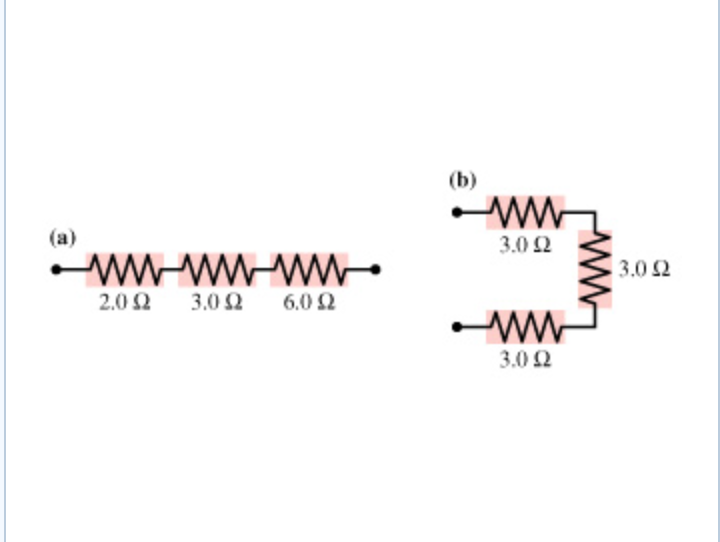What is the equivalent resistance of group (a) of resistors shown in the figure? (Figure 1) Express your answer using two significant figures. Req =   ?   Part B What is the equivalent resistance of group (b) of resistors shown in the figure? Express your answer using two significant figures.